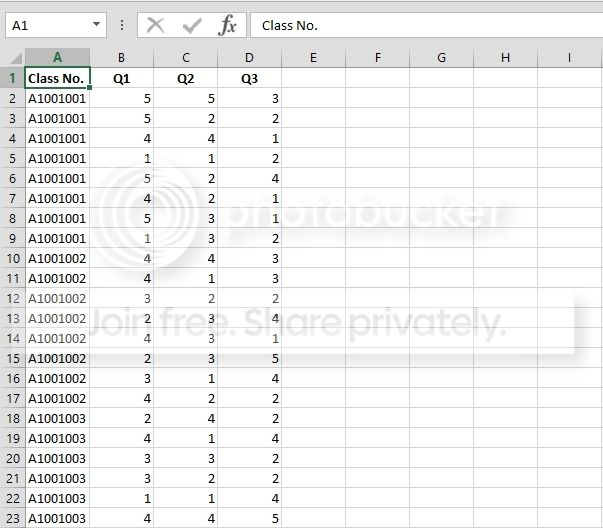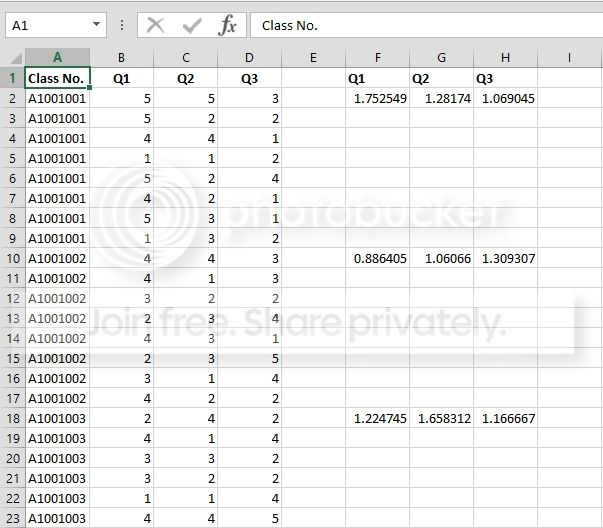## Wednesday 22 April 2015

### STDEVIF

Excel does not have a STDEVIF function. It's got other functions like COUNTIF(S), SUMIF(S) and AVERAGEIF(S), but is somewhat lacking when it comes to calculating conditional standard deviation.

If you have a lot of data, that can be a problem. Using array formulas or a UDF can slow things down. So let's focus on what's important, why not just get the calculation results? Forget using functions or VBA, why not both? (This applies to hard and soft tacos also!)

Here's my data. Note that the values in Column A are sorted. This is important.In my little code snippet down below,

Conditions (Class numbers in this case) are in Column A, so iCondCol = 1.

The values start in Column B, so iValsStartCol = 2.

The number of columns to calculate are 3 (Columns B:D), so iNumbOfCols = 3.

I'll put the results in Column F, so iEnterValsStartCol = 6

And I'll choose StDev for sFunctionType. I've also added Max for MAXIF and Min for MINIF, but feel free to add your own.

Update: Since I wrote this post, Microsoft launched Excel 2016 which includes MAXIF(S) and MINIF(S) functions. But like I say, this method is still very useful for a lot of other functions that don't have conditional variations yet! So for STDEVP, STDEV.S, STDEV.P, etc, substitute where necessary.

Here we go...

```Sub GetConditionalData()     Dim sFunctionType As String     Dim lHeaderRow As Long     Dim bAddHeaders As Boolean     Dim lStartRow As Long     Dim iCondCol As Integer     Dim iValsStartCol As Integer     Dim iNumbOfCols As Integer     Dim iEnterValsStartCol As Integer     Dim iCol As Integer     Dim rCell As Range     Dim rCond As Range     Dim rAddVal As Range     Dim rColumn As Range     Dim sTemp As String     Dim lCalc As Long          On Error Resume Next          'Change function type, columns, and headers as necessary     '********************************************************          'This code currently allows StDev, Max and Min     'Add your own function types as required (See code below)     sFunctionType = "StDev"              'Add column headers     bAddHeaders = True          'Which row has the headers?     lHeaderRow = 1          'Which row does the data start?     lStartRow = 2          'Which column has the conditional data?     iCondCol = 1          'Which column do the values start?     iValsStartCol = 2          'How many columns to loop through?     iNumbOfCols = 3          'Which column to enter the calculated values?     iEnterValsStartCol = 6          '********************************************************          Select Case sFunctionType                  'Add function types here         Case "StDev", "Max", "Min"                  Case Else                  MsgBox "You did not choose an included function.", vbExclamation, "Get Conditional Data"                  Exit Sub              End Select          With Application         lCalc = .Calculation         .Calculation = xlCalculationManual         .ScreenUpdating = False         .EnableEvents = False     End With          With ActiveSheet                  For Each rCell In Range(.Cells(lStartRow, iCondCol), .Cells(.Rows.Count, iCondCol).End(xlUp)).Cells                          With rCell                                  If rCond Is Nothing Then Set rCond = rCell                                  If .Value = .Offset(1).Value Then                                          Set rCond = Union(rCond, rCell.Offset(1))                                      Else                                          Set rCond = Nothing                                      End If                              End With                          If Not rCond Is Nothing Then                                  For Each rAddVal In rCond.Cells                                          For iCol = 1 To iNumbOfCols                                                  With Cells(rAddVal.Row, iEnterValsStartCol + iCol - 1)                                                          'You must add code for your function type here                             Select Case sFunctionType                                                                  Case "StDev"                                 .Value = WorksheetFunction.StDev(Range(rCond.Offset(, iValsStartCol - iCondCol + iCol - 1).AddressLocal))                                                                  Case "Max"                                 .Value = WorksheetFunction.Max(Range(rCond.Offset(, iValsStartCol - iCondCol + iCol - 1).AddressLocal))                                                                  Case "Min"                                 .Value = WorksheetFunction.Min(Range(rCond.Offset(, iValsStartCol - iCondCol + iCol - 1).AddressLocal))                                                                                              End Select                                                      End With                                              Next iCol                                      Next rAddVal                              Else                                  For iCol = 1 To iNumbOfCols                                          With Cells(rCell.Row, iEnterValsStartCol + iCol - 1)                                                  'You must add code for your function type here                         Select Case sFunctionType                                                          Case "StDev"                             .Value = WorksheetFunction.StDev(Range(rCell.Offset(, iValsStartCol - iCondCol + iCol - 1).AddressLocal))                                                          Case "Max"                             .Value = WorksheetFunction.Max(Range(rCell.Offset(, iValsStartCol - iCondCol + iCol - 1).AddressLocal))                                                          Case "Min"                             .Value = WorksheetFunction.Min(Range(rCell.Offset(, iValsStartCol - iCondCol + iCol - 1).AddressLocal))                                                      End Select                                              End With                                      Next iCol                              End If                      Next rCell                  'Get the Column headers if required         If bAddHeaders = True Then                          For iCol = 1 To iNumbOfCols                                  Cells(lHeaderRow, iEnterValsStartCol + iCol - 1).Value = Cells(lHeaderRow, iValsStartCol - iCondCol + iCol).Value                              Next iCol                      End If                  With Range(.Cells(lStartRow, iEnterValsStartCol), .Cells(.Rows.Count, iEnterValsStartCol).End(xlUp)).Resize(, iNumbOfCols)                          'Enter zeroes in blank cells (eg, calculating StDev was not possible)             .SpecialCells(xlCellTypeBlanks).Value = 0                          'Delete duplicates (comment out this code if you want to keep them)             '******************************************************************             For Each rColumn In .Columns                                  For Each rCell In rColumn.Cells                                          With rCell                                                  sTemp = Cells(.Row, iCondCol).Value                                                  If Cells(.Row, iCondCol).Offset(1).Value = sTemp Then .Offset(1).Value = ""                                              End With                                      Next rCell                              Next rColumn             '******************************************************************                      End With              End With          With Application         .Calculation = lCalc         .ScreenUpdating = True         .EnableEvents = True     End With          On Error GoTo 0 End Sub ```
And we're done.The code used the VBA version of STDEV (WorksheetFunction.StDev) to calculate the results, and put them in the appropriate column. So yeah, functions and VBA, Excel has fun for everyone.

Note the code deletes duplicates. I figure just one result per condition from each of the value columns is enough.

If you're using the data to print reports, like results per class, just use a LOOKUP function variant or MATCH/INDEX combination to find the relevant values.

How about STDEVIFS? Sure, just use suitable functions to get a unique string in a helper column, and refer to it instead. Then make sure it's sorted and you're good to go.

See you next time.